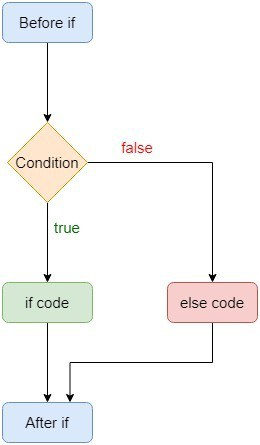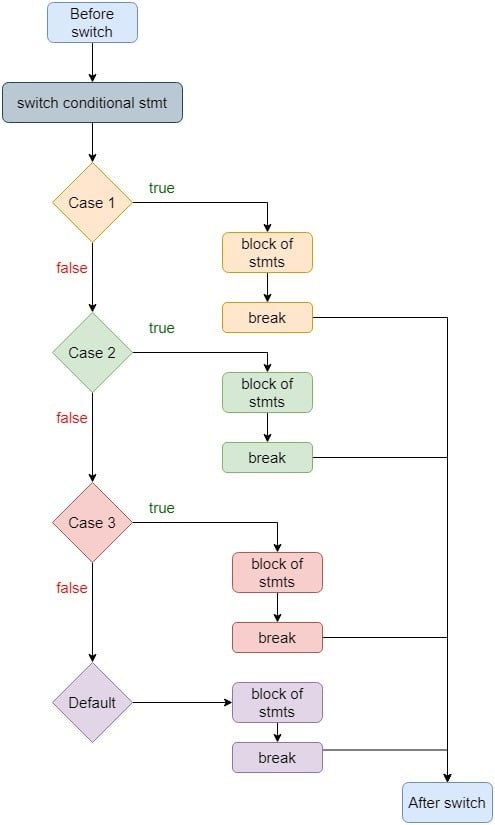## 1. Introduction

In this tutorial, we’ll study and compare two types of conditional statements: the if-else and switch statements.

## 2. Conditional Constructs in Computer Programming

We use conditional statements to execute a specific set of instructions based on a condition. This condition is usually a Boolean expression that evaluates to true or false:That is the case with the if-else statement. However, conditional logic may have more than two branches:In that case, we use switch statements.

## 3. Differences Between If-Else and Switch

Let’s now compare the two statements.

### 3.1. Readability of If-Else and Switch

A switch block is much more readable and maintainable than chained if-else statements. It’s easier to extend a switch case block than an if-else block because we have to evaluate all previous if-else conditions to correctly insert a new else block. In contrast, we can easily add and remove labels in the switch statement. So, switch statements make code significantly easier to change and maintain.

Multiple if-else branches are harder to read and understand, especially when they are nested and numerous. As a result, a chained if-else block is quite vulnerable to errors as we can easily miss an else statement. That can introduce bugs.

When it comes to code footprint, an if-else ladder can be written in less number of lines than a switch block. We don’t have to use break and default in case of an if-else block. However, we find that the if-else statement block is repetitive whereas the switch block is sequential.

### 3.2. Constant Case Label in Switch

For most modern programming languages such as C, C++, and Java, the switch conditional must know the values of each of its cases at compile time. This means that we can’t use variables as case values. We have to only use constant expressions for switch cases. However, there are few programming languages such as Swift that allow us to use variables and expressions in case statements.

On the other side of the coin, in an if-else block, the condition is evaluated when the code is executed, so it supports variables and expressions.

### 3.3. Falling Case Problem in Switch

The switch statement suffers from the problem of a falling case in most programming languages. This problem occurs when programmers forget to add a break statement in any individual case block. As a result, the code will execute that case block and then move to the very next case block to execute it too. That continues till the code finds a break statement or executes all the cases.

Let’s consider the following C code snippet. It will print 123Unknown value instead of just printing 1:

``````int main()
{
int value=1;

switch(value)
{
case 1:
printf("1");
case 2:
printf("2");
case 3:
printf("3");
default:
printf("Unknown value");
}
}``````

However, in Swift, there is no fall-through on switch statements, so we don’t need breaks to localize the flow.

### 3.4. The Internal Implementation of Switch

Most compilers implement the switch construct as a jump table. A jump table is an abstract data structure that is used by modern days compilers to transfer the flow control to another location. The actual implementation of the jump table can differ based on the number of cases. When there are only a handful of cases, it can be in form of a dictionary or a map, whereas for a large number of cases, it’s usually a hash table.

The compiler matches the case with the switch expression and then executes it by making a jump in the table. Thus, our switch statement will be much more efficient than if-else when our case labels are close together. This is so because most of the jumps will be sequential in memory. To make a jump, we simply add a value to the base program counter that points to the table base.

### 3.5. Switch Internal Example

We can better understand it with an example. This is a simple switch block:

``````switch (i)
{
case 1: printf("case 1"); break;
case 11: printf("case 11"); break;
case 111: printf("case 111"); break;
}
``````

For this switch statement, our compiler can generate the following crude function calls for each case:

``````void case1() { printf("case 1"); }
void case11() { printf("case 11"); }
void case111() { printf("case 111"); }``````

Then, it will use a function pointer to make the proper call at run time:

``````typedef void (*pswitchfunccallback)(void);
pswitchfunccallback func_array = {case1, case11, case111};

if ((unsigned)i<111)
func_array[i]();``````

This will have the time complexity ofwhereis the number of distinct case blocks. The address of each of the functions is stored in the jump table and is accessed by an optimized hash function.

### 3.6. If-Else Internal Implementation

On the other hand, most of the compilers construct a binary tree for an if-else conditional block. For a typical if-else, block, the compiler converts it into a list of tokens and then creates an abstract syntax tree out of those tokens. This tree is later evaluated based on comparisons to select a particular branch.

So, this works by doing the inherently slow comparisons.

### 3.7. Speed of If-Else and Switch

In general, we find the execution time of a switch case lower than that of an if-else block in the case where the value set is relatively small and each value is equally likely to occur. In the case the value set is large and input values aren’t uniform, the if-else blocks perform better if the most frequent values are covered by conditions early in the chain.

In practice, however, the difference between these two statements’ execution times may be negligible.